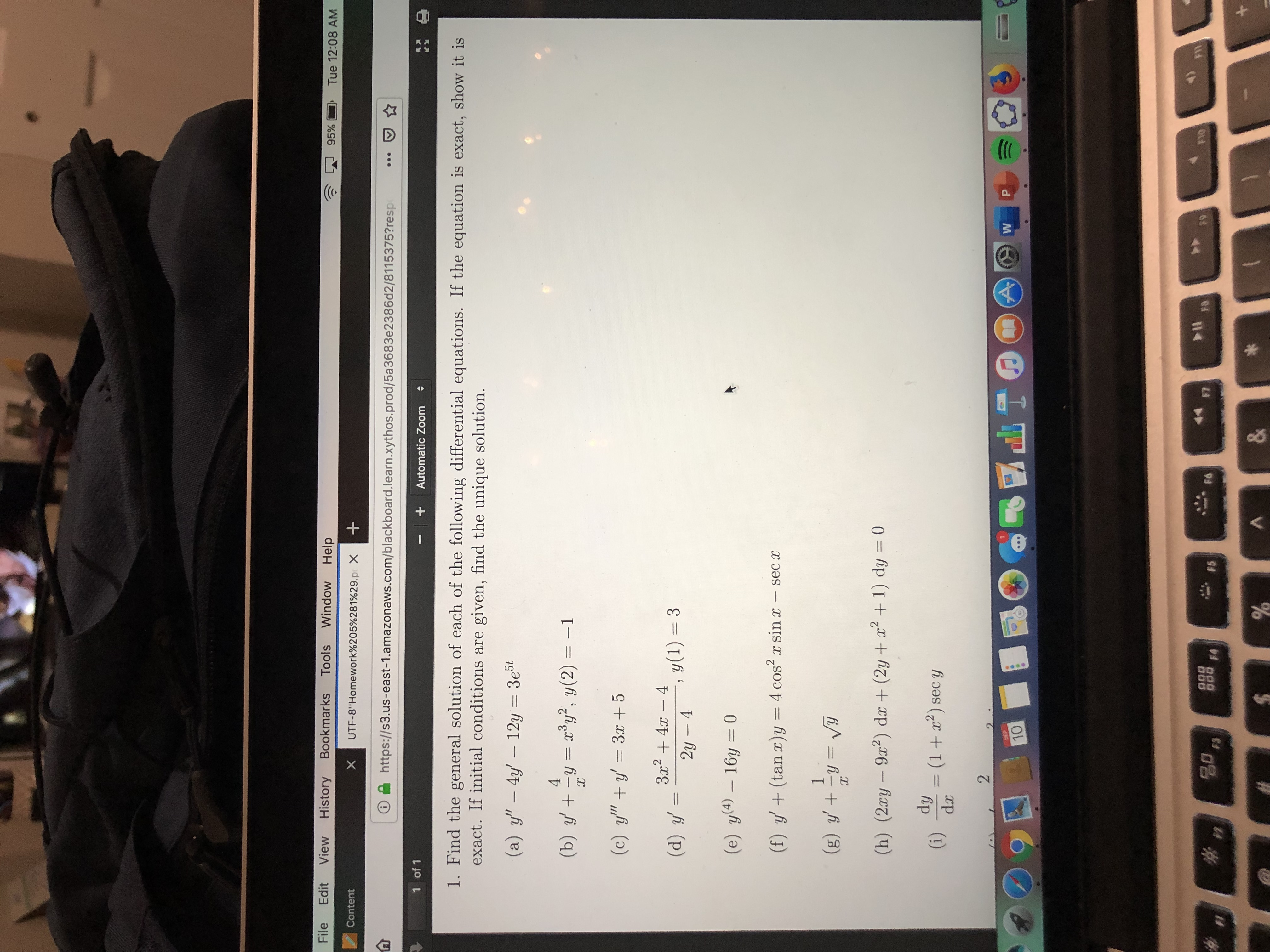# FileEditViewHistoryBookmarksToolsWindowHelp95%Tue 12:08 AMUTF-8"Homework%205%281%29. poX+ContentXhttp:://s3.us-east-1.amazonaws.com/blackboard.learn.xythos.prod/5a3683e2386d2/8115375? resp1 of 1Automatic Zoom1. Find the general solution of each of the following differential equations. If the equation is exact, show it isexact. If initial conditions are given, find the unique solution.(a) y"- 4y 12y 3e5t4y, y(2) = -1(b) yyX(c) y"y 3x + 5324-4(d) y, y(1) = 32y 4(e) y(4)-16y 0(f) y(tan r)y = 4 cos2sec1(g) y y VYIC(h) (2ry -9r2) dæ (2y+ x21) dy 0dy(1+ sec y(i)dx2PGEP10FIFYOF8F7O00 FAFóF5&

Question
2 views

bhelp_outlineImage TranscriptioncloseFile Edit View History Bookmarks Tools Window Help 95% Tue 12:08 AM UTF-8"Homework%205%281%29. poX + Content X http:://s3.us-east-1.amazonaws.com/blackboard.learn.xythos.prod/5a3683e2386d2/8115375? resp 1 of 1 Automatic Zoom 1. Find the general solution of each of the following differential equations. If the equation is exact, show it is exact. If initial conditions are given, find the unique solution. (a) y"- 4y 12y 3e5t 4 y, y(2) = -1 (b) yy X (c) y"y 3x + 5 324-4 (d) y , y(1) = 3 2y 4 (e) y(4)-16y 0 (f) y(tan r)y = 4 cos2 sec 1 (g) y y VY IC (h) (2ry -9r2) dæ (2y+ x21) dy 0 dy (1+ sec y (i) dx 2 P GEP 10 FI FYO F8 F7 O00 FA Fó F5 & fullscreen
check_circle

Step 1

The given equation is of the form y+ P y = Q yn , n is not equal to 0,1. Where P and Q are constants or functions of x (not of y) and n is a constant except 0 and 1 , is called Bernoulli’s differential equation.

So, the given differential equation:

y+ (4/x) y = x3y2 , y(2)=-1    ......(1)

For general solution of the given differential equation:

Multiply by y-2 therefore the differential equation:

y-2 (y+ (4/x) y) = y-2 (x3y2

y-2 y+ (4 /x) y-1 = x3 ......(2)

Let 1/y = v    .......(3)

Differentiate (3) with respect to x, gives:

-(1/y2) (dy /dx) =dv/dx

Equation (2) reduced in the form:

-(dv/dx) +(4/x) v =x3

(dv/dx) -(4/x) v = - x3

Which is the standard form of the linear equation in v.

Its integration factor is:

Step 2

Its solution is of the form:

Step 3

The differential equation M(x,y) dx + N(x,y) dy...

### Want to see the full answer?

See Solution

#### Want to see this answer and more?

Solutions are written by subject experts who are available 24/7. Questions are typically answered within 1 hour.*

See Solution
*Response times may vary by subject and question.
Tagged in

### Math